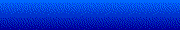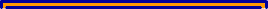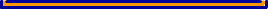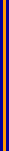HomeTechnologyComparisonStudiesInvestmentPreferential TreatmentCalculatorsCollaborationContactThe two utility applications shown below allow ad hoc calculations for the electrical output limits of a moderately sized Horizon platform.  A platform is composed of several modules - 96 for the purposes of these calculators.  The first calculator performs its operations using metric values.  The second utilizes traditional English units.  We provided both varieties so that users could run the numbers using the measurement system with which they are most accoustomed.

### Calculations Using the Metric System

Each module contains six floats.  Each float displaces 2,388 kilograms of water.  The floats are designed so that at rest in calm water they are half submerged.  Therefore, they can exert 2,388 killograms of force in both rising and falling waves.  The floats are 1.2 meters tall.  This is pertinent because the application reduces the vertical travel of the floats by this amount when performing the calculations.  In order to generate maximum horsepower, the floats must be almost completely immersed in rising waves, and almost completely out of the water in falling waves.  Accordingly, if a swell height of 3 meters is input, the application will reduce it by 1.2, and use 1.8 instead.  This mimics the real world motion of the floats as they work against the resistance of the generator.

The following bulleted list defines the calculated values produced by the application:

• The meters per second is the swell height less the float height, multiplied by two and divided by the period.
[i.e., (SwellHeight - 1.2) * 2 / SwellPeriod]
• The horsepower generated per module is a function of the six float weights multiplied by the meters per second divided by 76 (the conversion factor for metric horsepower).
[i.e., 2,388 * 6 * FeetPerSecond / 76]
• The kilowatts per module is horsepower multiplied by 0.7355 (the conversion factor for killowatts).
[i.e., Horsepower * 0.7355]
• The total platform killowatts is the killowatts per module multiplied by the number of modules (96).
[i.e., KillowattsPerModule * 96]
• The annual revenue is the revenue per megawatt input multiplied by the total platform killowatts divided by 1000 (the conversion factor for megawatts), multiplied by the number of hours in a day (24), multiplied by the number of days in a year (365).
[i.e., RevenuePerMegawatt * TotalPlatformKillowatts / 1000 * 24 * 365]

Note: All of the calculated values are rounded for display.

The Flash player (version 8 or later) must be installed in order to view/use this web-site.

### Calculations Using the English System

Each module contains six floats.  Each float displaces 5,265 pounds of water.  The floats are designed so that at rest in calm water they are half submerged.  Therefore, they can exert 5,265 pounds of force in both rising and falling waves.  The floats are 4 feet tall.  This is pertinent because the application reduces the vertical travel of the floats by this amount when performing the calculations.  In order to generate maximum horsepower, the floats must be almost completely immersed in rising waves, and almost completely out of the water in falling waves.  Accordingly, if a swell height of 10 is input, the application will reduce it by 4, and use 6 instead.  This mimics the real world motion of the floats as they work against the resistance of the generator.

The following bulleted list defines the calculated values produced by the application:

• The feet per second is the swell height less the float height, multiplied by two and divided by the period.
[i.e., (SwellHeight - 4) * 2 / SwellPeriod]
• The horsepower generated per module is a function of the six float weights multiplied by the feet per second divided by 550 (the conversion factor for horsepower).
[i.e., 5,265 * 6 * FeetPerSecond / 550]
• The kilowatts per module is horsepower multiplied by 0.7457 (the conversion factor for killowatts).
[i.e., Horsepower * 0.7457]
• The total platform killowatts is the killowatts per module multiplied by the number of modules (96).
[i.e., KillowattsPerModule * 96]
• The annual revenue is the revenue per megawatt input multiplied by the total platform killowatts divided by 1000 (the conversion factor for megawatts), multiplied by the number of hours in a day (24), multiplied by the number of days in a year (365).
[i.e., RevenuePerMegawatt * TotalPlatformKillowatts / 1000 * 24 * 365]

Note: All of the calculated values are rounded for display.

The Flash player (version 8 or later) must be installed in order to view/use this web-site.